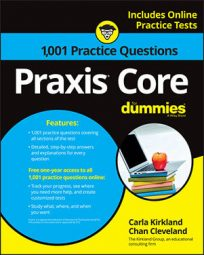##### Praxis Core: 1,001 Practice Questions For DummiesRate problems on the Praxis Core exam will often appear as word problems, so you'll have to read them carefully to make sure you know what you're being asked to find.

In the following practice questions, you're first given two moving trains and you have to calculate the time required for them to travel a given distance; then you're given a runner's distance traveled in a given amount of time, and you have to find his average speed—after doing some distance and time conversions.

## Practice questions

1. Two trains start at the same place and move in opposite directions. One train moves at a constant speed of 56 kilometers per hour, and the other train moves at a constant speed of 72 kilometers per hour. How many hours will it take for the trains to be 729.6 kilometers apart?

A. 10.13 B. 13 C. 601.6 D. 5.7 E. 0.175

2. Herschel ran 40 yards in 4.2 seconds. What was his average speed in feet per minute, rounded to the nearest hundredth?

A. 28.57 feet per minute B. 571.43 feet per minute C. 8.40 feet per minute D. 9.52 feet per minute E. 1,714.29 feet per minute

1. The correct answer is Choice (D). The speed (rate) at which the trains are moving apart is the sum of 56 kilometers per hour and 72 kilometers per hour, or 128 kilometers per hour. The distance in question is 729.6 kilometers. The problem asks how long the trains take to cover this distance. You can use the distance formula to determine the time. Substitute the known values in for the variables and solve for t:

Because the calculations involve kilometers per hour, the value of t is in hours.

2. The correct answer is Choice (E).

The question asks for a rate in feet per minute, so convert 40 yards to feet and 4.2 seconds to minutes:

Now, write a ratio of those quantities and reduce it:

Round to the nearest hundredth, which is two places after the decimal point. The third place after the decimal point is 5, so round up:

The correct answer, rounded to the nearest hundredth, is 1,714.29 feet per minute.